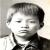# 12个有趣的C语言问答热

junwong 发布于 2012/09/06 14:54

1，gets() 方法
Q:以下代码有个被隐藏住的问题，你能找到它吗？
```#include<stdio.h>

int main(void)
{
char buff;
memset(buff,0,sizeof(buff));

gets(buff);

printf("\n The buffer entered is [%s]\n",buff);

return 0;
}```

A:这个不显眼的问题就是使用了 gets() 方法。此方法接受一个string类型参数，但是却没有检测此数值是否 有足够的空间来拷贝数据。所以这里我们一般用 fgets() 方法将来的更好。

2，strcpy() 方法
Q:密码防护是很基本的功能，看看能否搞定下面这段代码?
```#include<stdio.h>

int main(int argc, char *argv[])
{
int flag = 0;
char passwd;

memset(passwd,0,sizeof(passwd));

strcpy(passwd, argv);

if(0 == strcmp("LinuxGeek", passwd))
{
flag = 1;
}

if(flag)
{
}
else
{
printf("\n Incorrect passwd \n");

}
return 0;
}```

3，main() 方法的返回类型
Q:请问下面这段代码能否通过编译？如果能的话，那么这段代码中隐含什么问题吗？
```#include<stdio.h>

void main(void)
{
char *ptr = (char*)malloc(10);

if(NULL == ptr)
{
printf("\n Malloc failed \n");
return;
}
else
{
// Do some processing

free(ptr);
}

return;
}```

A:答案是代码能通过编译，但是会留下针对main()方法的返回类型的警告。main()方法的真正返回类型应该为'int'而非'void'。这是因为'int'返回类型能够让程序返回状态值。尤其是当这段程序作为其他应用的附属程序时这个状态值将更加重要。

4，内存泄露
Q:请问以下代码有内存泄露吗？
```#include<stdio.h>

void main(void)
{
char *ptr = (char*)malloc(10);

if(NULL == ptr)
{
printf("\n Malloc failed \n");
return;
}
else
{
// Do some processing
}

return;
}```

A:好，虽然上面的代码没有对指针 ptr 进行内存释放，但实际上即使是程序结束也不会造成内存泄露，因为当程序结束时所有一开始被占据的内存就全部清空了。但如果上面这段代码是在 while 循环里面那将会造成严重的问题。

Note: 如果你需要了解更多关于内存泄露的问题以及如何使用工具检测内存泄露，你可以参考这篇文章 Valgrind

5，free() 方法
Q:以下代码当用户输入'freeze'时会奔溃，而如果输入'zebra'则运行正常，这是为什么？
```#include<stdio.h>

int main(int argc, char *argv[])
{
char *ptr = (char*)malloc(10);

if(NULL == ptr)
{
printf("\n Malloc failed \n");
return -1;
}
else if(argc == 1)
{
printf("\n Usage  \n");
}
else
{
memset(ptr, 0, 10);

strncpy(ptr, argv, 9);

while(*ptr != 'z')
{
if(*ptr == '')
break;
else
ptr++;
}

if(*ptr == 'z')
{
printf("\n String contains 'z'\n");
// Do some more processing
}

free(ptr);
}

return 0;
}```

A:问题的根源是因为代码在while循环中改变了 ptr 指针的地址。当输入为'zebra'时，while循环甚至在执行 第一遍前就结束了，所以free()释放的内存地址就是一开始malloc()分配的地址。但是当输入'freeze'时， ptr记录的地址在while循环中被更改，因为将会是错误的地址传递到free()方法中引起崩溃。

6，atexit with _exit
Q:在以下代码，atexit()方法并没有被调用，你知道为什么吗？
```#include<stdio.h>

void func(void)
{
printf("\n Cleanup function called \n");
return;
}

int main(void)
{
int i = 0;

atexit(func);

for(;i<0xffffff;i++);

_exit(0);
}```

A:这是因为使用了 _exit() 方法。此方法并没有调用清除数据相关的方法，比如 atexit()等。

7，void* 与 C 结构体
Q:能否设计一个方法接受任意类型的参数然后返回整数？同时是否有办法传递多个这样的参数？
A：一个能接受任意类型参数的方法像下面这个样子：
` int func(void *ptr)`

8，* 与 ++ 操作符
Q:以下代码将输出什么？为什么？
```#include<stdio.h>

int main(void)
{
char *ptr = "Linux";
printf("\n [%c] \n",*ptr++);
printf("\n [%c] \n",*ptr);

return 0;
}```

A:以上的输出将是:
```[L]

[i]```

9，Making changes in Code segment
Q:以下代码运行时一定会崩溃，你能说出原因吗？
```#include<stdio.h>

int main(void)
{
char *ptr = "Linux";
*ptr = 'T';

printf("\n [%s] \n", ptr);

return 0;
}```

A:这是因为，通过 *ptr = 'T'，此行代码尝试更改只读内存存储的字符串'Linux'。此操作当然行不通所以才会造成崩溃。

10，Process that changes its own name
Q:你能否写一个程序在它运行时修改它的名称？
A:以下代码可以完成
```#include<stdio.h>

int main(int argc, char *argv[])
{
int i = 0;
char buff;

memset(buff,0,sizeof(buff));

strncpy(buff, argv, sizeof(buff));
memset(argv,0,strlen(buff));

strncpy(argv, "NewName", 7);

// Simulate a wait. Check the process
// name at this point.
for(;i<0xffffffff;i++);

return 0;
}```

11，局部变量的返回地址
Q:下面的代码有问题吗？如果有，如何修改？
```#include<stdio.h>

int* inc(int val)
{
int a = val;
a++;
return &a;
}

int main(void)
{
int a = 10;

int *val = inc(a);

printf("\n Incremented value is equal to [%d] \n", *val);

return 0;
}```

A:虽然上面的代码有时运行会很好，但是在方法 inc() 中有很严重的隐患。当inc()方法执行后，再次使用局部变量的地址就会造成不可估量的结果。解决之道就是传递变量a的地址给main()。

12，处理 printf() 参数
Q:以下代码输出请问是什么？
```#include<stdio.h>

int main(void)
{
int a = 10, b = 20, c = 30;

printf("\n %d..%d..%d \n", a+b+c, (b = b*2), (c = c*2));

return 0;
}```

A:输出将是
`110..40..60`

0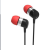#### 引用来自“vingzhang”的答案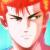00@zwx1205 我不是说不能编译，是表现跟文章描述不符
z

0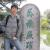0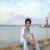C语言什么时候有string类型了？